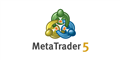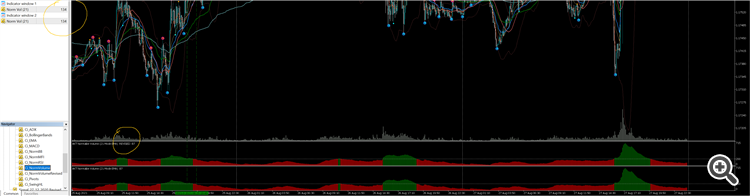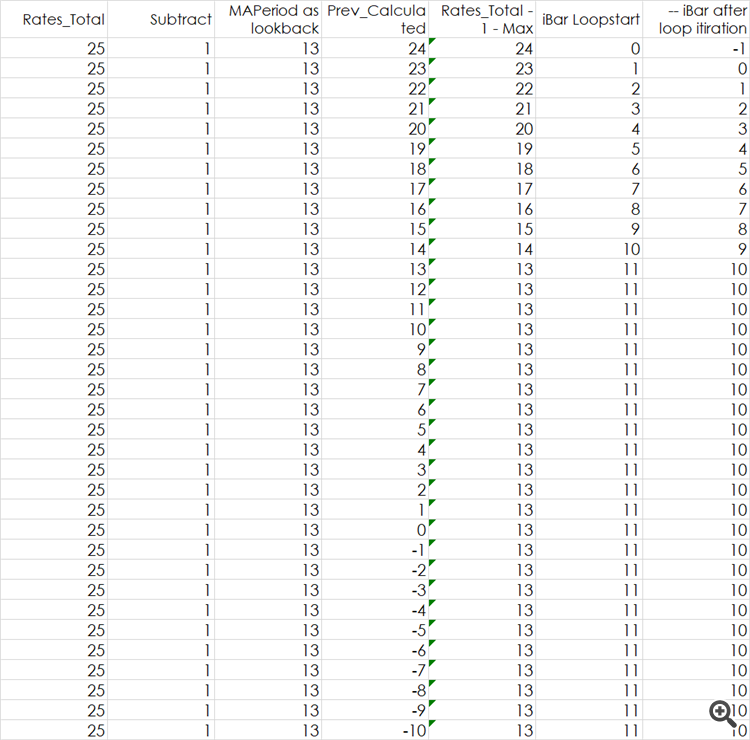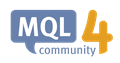# Volume Indicator is TOO SLOW! Seeking help to find root cause of it.

[Deleted]

UPDATE NOTE: Finally the issue have been sorted out.

Hi

Wishing Happy Weekend to all.

I am struggling hard to create an optimized "Normalized Volume Indicator".

I have used few other methods too including MAOnBuffer() functions.

Following code is my latest attempt, the same code I am using in Price EMA and is working fine even with 5EMAs calculated in a single indicator file.

I am not sure is the tick_volume[] or the Histogram is causing the below one to be slow. As all other calculations are same as in my EMA Indicator.

Your help will be highly appreciated.

```//+----------------------------------------------------------------------------------------------------------+
//| Ci_NormVolume.mq5
//+----------------------------------------------------------------------------------------------------------+
#property description "Normalized Volume"

#property indicator_separate_window
#property indicator_buffers 2
#property indicator_plots   1
#property indicator_color1  clrGreen,clrRed
//+----------------------------------------------------------------------------------------------------------+
//| Define Input Parameters
//+----------------------------------------------------------------------------------------------------------+
input int                   Inp_MAPeriod      = 21;           // Averaging Period
input ENUM_APPLIED_VOLUME   Inp_AppliedVolume = VOLUME_TICK;  // Applied Volume Type
input int                   Inp_Threshold     = 200;          //
//+----------------------------------------------------------------------------------------------------------+
//| Global Variables
//+----------------------------------------------------------------------------------------------------------+
int    vMAPeriod;
//+----------------------------------------------------------------------------------------------------------+
//| Define Indicator Buffers for Data & Arrays for Indicator Calculation
//+----------------------------------------------------------------------------------------------------------+
double Buffer_NormVolume[];
double Buffer_Colors[];
//+----------------------------------------------------------------------------------------------------------+
//| Custom indicator initialization function
//+----------------------------------------------------------------------------------------------------------+
int OnInit()
{
//+--------------------------------------------------------------------------------------------------------+
//| Check Input Parameter Values and set them to Minimum Recommended, if required
//+--------------------------------------------------------------------------------------------------------+
if(Inp_MAPeriod < 2)
{
vMAPeriod = 55;
PrintFormat("Parameter Inp_MAPeriod=%d. Min Recommended value=%d used for calculations.",Inp_MAPeriod,vMAPeriod);
}
else
vMAPeriod = Inp_MAPeriod;
//+--------------------------------------------------------------------------------------------------------+
//| Indicator SubWindow common 'properties' (used instead of #property)
//+--------------------------------------------------------------------------------------------------------+
IndicatorSetString(INDICATOR_SHORTNAME,"AKT Normalize Volume ("+(string)vMAPeriod+" Mode EMA) ");
IndicatorSetInteger(INDICATOR_DIGITS,0);          // Set n digits for Normalize Volume
IndicatorSetDouble(INDICATOR_MINIMUM,0.00);
IndicatorSetInteger(INDICATOR_HEIGHT,125);
//--- LEVELS
IndicatorSetInteger(INDICATOR_LEVELS,0,clrSilver);
IndicatorSetDouble(INDICATOR_LEVELVALUE,0,Inp_Threshold);
//+--------------------------------------------------------------------------------------------------------+
//| Indicator Buffers mapping / binding
//+--------------------------------------------------------------------------------------------------------+
SetIndexBuffer(0,Buffer_NormVolume,INDICATOR_DATA);
SetIndexBuffer(1,Buffer_Colors,INDICATOR_COLOR_INDEX);
//+--------------------------------------------------------------------------------------------------------+
//| Plot Indicator Labels in SubWindow (used instead of #property)
//+--------------------------------------------------------------------------------------------------------+
PlotIndexSetString(0,PLOT_LABEL,"Norm Vol ("+string(vMAPeriod)+")");
PlotIndexSetInteger(0,PLOT_DRAW_TYPE,DRAW_COLOR_HISTOGRAM);
PlotIndexSetInteger(0,PLOT_LINE_STYLE,STYLE_SOLID);
PlotIndexSetInteger(0,PLOT_LINE_WIDTH,2);
PlotIndexSetDouble(0,PLOT_EMPTY_VALUE,EMPTY_VALUE);
//--- indexes draw begin settings
//PlotIndexSetInteger(0,PLOT_DRAW_BEGIN,vMAPeriod);
//---
return(0);     // required when int OnInit(), originally void OnInit()
}
//+----------------------------------------------------------------------------------------------------------+
//| MAIN Iteration function for Custom Normalize Volume Indicator
//+----------------------------------------------------------------------------------------------------------+
int OnCalculate(const int       rates_total,
const int       prev_calculated,
const datetime  &time[],
const double    &open[],
const double    &high[],
const double    &low[],
const double    &close[],
const long      &tick_volume[],
const long      &volume[],
{
//ulong start = GetMicrosecondCount();                      // CALCULATION START TIME
ArrayResize(Buffer_NormVolume,rates_total);
double vSmoothing = 2.0/(1.0 + Inp_MAPeriod);
//+--------------------------------------------------------------------------------------------------------+
//| Loop to FILL Applied Volume values into Buffer_Volume
//+--------------------------------------------------------------------------------------------------------+
for(int i=(int)MathMax(prev_calculated-1,0); i < rates_total; i++)
{
double vVolume;
if(Inp_AppliedVolume == VOLUME_TICK)
vVolume = (double)tick_volume[i];
else
vVolume = (double)volume[i];

if(i < 2)   // avoid Zero bar, as it keeps changing and affect the Average Value
{
Buffer_NormVolume[i] = (vVolume);
continue;
}
Buffer_NormVolume[i] = Buffer_NormVolume[i-1] + vSmoothing * (vVolume - Buffer_NormVolume[i-1]);
Buffer_Colors[i]     = (Buffer_NormVolume[i] < Inp_Threshold ? 1:0);
}
//---
//ulong finish = GetMicrosecondCount();                   // CALCULATION END TIME
//PrintFormat("%s in %s took %.1f ms",__FUNCTION__,__FILE__,(finish-start)/1000); // Get Calculation time used by the indicator
//--- OnCalculate done, return new prev_calculated.
return(rates_total);
} // END Of Ci_NormVolume indicator calculation
//+----------------------------------------------------------------------------------------------------------+
```Volumes - Volume Indicators - MetaTrader 5 Help
For the Forex market, Volumes is the indicator of the number of price changes within each period of a selected timeframe. For stock symbols this is...

I cannot see why it is slow, but your "zero bar" is at the wrong end of the array.

1. Dominik Egert  your "zero bar" is at the wrong end of the array.

You must set as-series or non-series to your arrays and buffers, so they match your loop.

2. ```
if(i < 2)   // avoid Zero bar, as it keeps changing and affect the Average Value
{
Buffer_NormVolume[i] = (vVolume);
continue;```

Bar zero is not changing. You have to avoid it or indicator crashes at

`vVolume - Buffer_NormVolume[i-1]);`
[Deleted]
William Roeder #:
1. You must set as-series or non-series to your arrays and buffers, so they match your loop.

2. Bar zero is not changing. You have to avoid it or indicator crashes at

Hi William

Thanks for your reply. for last couple of hours I have been juggling to implement your suggestions.

First I tried the Count Down method, and had a strange results as output was having sort of Bar Shift effect in display equal to MAPeriod!!! However, there was not much improvement still in the speed of Indicator.

Finally I tried the Count Up method, in the way it was suggested on your other post.

Well with this, at least the indicator is shown correctly however the cause of speed still remains a mystery. I have to run profiler but that also did not helped much.

Below is the REVISED Code (relevant section)
, image of both version of Indicator returning same values and profiler report.

Look forward from masters, to help me out.

regards.

```//+----------------------------------------------------------------------------------------------------------+
//| MAIN Iteration function for Custom Normalize Volume Indicator
//+----------------------------------------------------------------------------------------------------------+
int OnCalculate(const int       rates_total,
const int       prev_calculated,
const datetime  &time[],
const double    &open[],
const double    &high[],
const double    &low[],
const double    &close[],
const long      &tick_volume[],
const long      &volume[],
{
/******* Revision: Suggestion to set false while Count UP ******/
ArraySetAsSeries(Buffer_NormVolume,false);
ArraySetAsSeries(Buffer_Colors,false);
double vSmoothing = 2.0/(1.0 + Inp_MAPeriod);
//+--------------------------------------------------------------------------------------------------------+
//| Loop to FILL Applied Volume values into Buffer_Volume
//+--------------------------------------------------------------------------------------------------------+
//for(int i=(int)MathMax(prev_calculated-1,0); i < rates_total; i++)
/******* Revision: Suggestion to refraze for..loop ******/
for(int i = MathMax(vMAPeriod,prev_calculated); i < rates_total; i++)
{
double vVolume;
if(Inp_AppliedVolume == VOLUME_TICK)
vVolume = (double)tick_volume[i];
else
vVolume = (double)volume[i];

if(i < 2)
{
Buffer_NormVolume[i] = (vVolume);
continue;
}
Buffer_NormVolume[i] = Buffer_NormVolume[i-1] + vSmoothing * (vVolume - Buffer_NormVolume[i-1]);
Buffer_Colors[i]     = (Buffer_NormVolume[i] < Inp_Threshold ? 1:0);
}
//--- OnCalculate done, return new prev_calculated.
return(rates_total-1);  // REVISION:  Recalculate current bar next tick.
} // END Of Ci_NormVolume indicator calculation
//+----------------------------------------------------------------------------------------------------------+```If I am not wrong, returning rates_total - 1 will not work, as the calculations will never end.

If prev_calculated equals rates_total, you are on the most current bar.

You need to take extra measure to update the most current bars value.

Either subtract one from prev_calculated when it's equal to rates_total or have some other logic to update most current bar. (Ie skip the current bar and wait for it to be the last in history.)

Dominik Egert #: If I am not wrong, returning rates_total - 1 will not work, as the calculations will never end.
1. You are wrong. Indicators never end. What they return is irrevalent.
2. If you return rates_total - 1, you do not have to check prev_calculated to adjust starting position. See How to do your lookbacks correctly #9#14 & #19.

[Deleted]
Dominik Egert #:
If I am not wrong, returning rates_total - 1 will not work, as the calculations will never end.

If prev_calculated equals rates_total, you are on the most current bar.

You need to take extra measure to update the most current bars value.

Either subtract one from prev_calculated when it's equal to rates_total or have some other logic to update most current bar. (Ie skip the current bar and wait for it to be the last in history.)

Thans Dominik.

Well it did not worked me :)

[Deleted]
William Roeder #:
1. You are wrong. Indicators never end. What they return is irrevalent.
2. If you return rates_total - 1, you do not have to check prev_calculated to adjust starting position. See How to do your lookbacks correctly #9#14 & #19.

Thanks William

I tried but could not figure out why it not worked for me.

[Deleted]
Anil Varma - #:

Thanks William

I tried but could not figure out why it not worked for me.

Hi William

Just trying to understand the logic behind

**********************************************************************************************************

for(int iBar = rates_total-1-MathMax(lookback, prev_calculated); iBar >= 0; --iBar){

// Timeseries and Indicators Access uses index=iBar

}

return rates_total-1; // Recalculate current bar next tick.

**********************************************************************************************************

When I tried to put it mathematically in excel sheet, I am not convinced with this. May be you can please through some lights ...Timeseries and Indicators Access - MQL4 Reference
• docs.mql4.com
Timeseries and Indicators Access - MQL4 Reference

Anil Varma - #: Just trying to understand the logic behind

``` for(int iBar = rates_total-1-MathMax(lookback, prev_calculated); iBar >= 0; --iBar){
⋮
}
return rates_total-1; // Recalculate current bar next tick.```

1. First run prev_calculated is zero, loop runs from rates_total - 1 - lookback to zero. You can access iBar + (lookback-1) and not exceed rates_total-1.
2. If you return rates_total, next run is from  rates_total - 1 - rates_total. You said bar zero is done, so loop does nothing. #11
3. If you instead return rates_total - 1. Next run is rates_total - 1 - (rates_total - 1) or zero. Loop reprocesses bar zero's new tick.
4. If a new bar starts, rates_total increments (prev_calculated does not,) and next run is new_rates_total - 1 - (old_rates_total - 1) or one. Loop reprocesses last change of bar one and new tick of bar zero.
5. Your spreadsheet is bogus, you are counting down in the code.
[Deleted]
William Roeder #:
1. First run prev_calculated is zero, loop runs from rates_total - 1 - lookback to zero. You can access iBar + (lookback-1) and not exceed rates_total-1.
2. If you return rates_total, next run is from  rates_total - 1 - rates_total. You said bar zero is done, so loop does nothing. #11
3. If you instead return rates_total - 1. Next run is rates_total - 1 - (rates_total - 1) or zero. Loop reprocesses bar zero's new tick.
4. If a new bar starts, rates_total increments (prev_calculated does not,) and next run is new_rates_total - 1 - (old_rates_total - 1) or one. Loop reprocesses last change of bar one and new tick of bar zero.
5. Your spreadsheet is bogus, you are counting down in the code.

Thanks for explanation William.

need some time to digest it :)

Reason: